### Home > A2C > Chapter Ch13 > Lesson 13.1.3 > Problem13-46

13-46.
1. Solve each equation for 0°θ ≤ 360°, or if you prefer, 0 ≤ θ ≤ 2π. You may need your calculator, but remember that your calculator only gives one answer. Homework Help ✎

1. sin(θ) = 0.5

2. cos(θ) = −0.5

3. 4tan(θ) − 4 = 0

4. 3sin2(θ) = 1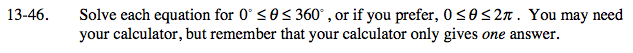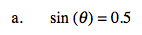$30\degree \text{ and }?\,\,\,\,\,\,\,\,\,\frac{\pi}{6}\text{ and }?$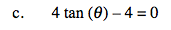tan(θ) = 1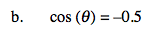$120\degree \text{ and }?\,\,\,\,\,\,\,\,\frac{2\pi}{3}\text{ and }?$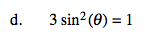$\text{sin(\theta) = \pm\frac{\sqrt{3}}{3}}$